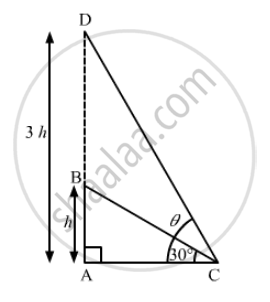Advertisement Remove all ads

# The Angle of Elevation of the Top of a Tower at a Point on the Ground is 30º. What Will Be the Angle of Elevation, If the Height of the Tower is Tripled? - Mathematics

Sum

The angle of elevation of the top of a tower at a point on the ground is 30º. What will be the angle of elevation, if the height of the tower is tripled?

Advertisement Remove all ads

#### SolutionLet the height of the tower AB be h units.

Suppose C is a point on the ground such that ∠ACB=30°

In right ∆ACB,

tan 30°= (AB)/(AC)

⇒ 1/sqrt3=h/(AC)

⇒ AC=sqrt3h                  ................(1)

Let the angle of elevation of the top of the tower at C be θ, if the height of the tower is tripled.
New height of the tower, AD = 3h units

In right ∆ACD,

tan θ= (AD)/(AC)

⇒ tan θ =(3h)/(AC)

⇒ tan θ= (3h)/sqrt(3h)=sqrt3           [from (1)]

⇒ tan θ= tan 60°

⇒ θ=60°

Hence, the required angle of elevation is 60º.

Is there an error in this question or solution?
Advertisement Remove all ads

#### APPEARS IN

RD Sharma Class 10 Maths
Chapter 12 Trigonometry
Q 8 | Page 41
Advertisement Remove all ads

#### Video TutorialsVIEW ALL 

Advertisement Remove all ads
Share
Notifications

View all notifications

Forgot password?
Course